# Neural networks

In this lesson, we will explore multilayer perceptrons (MLPs) which are a basic type of neural network. We'll first motivate non-linear activation functions by trying to fit a linear model (logistic regression) on our non-linear spiral data. Then we'll implement an MLP using just NumPy and then with PyTorch.

## Overview

Our goal is to learn a model $$\hat{y}$$ that models $$y$$ given $$X$$ . You'll notice that neural networks are just extensions of the generalized linear methods we've seen so far but with non-linear activation functions since our data will be highly non-linear.$z_1 = XW_1$
$a_1 = f(z_1)$
$z_2 = a_1W_2$
$\hat{y} = softmax(z_2)$

Variable Description
$$N$$ total numbers of samples
$$D$$ number of features
$$H$$ number of hidden units
$$C$$ number of classes
$$W_1$$ 1st layer weights $$\in \mathbb{R}^{DXH}$$
$$z_1$$ outputs from first layer $$\in \mathbb{R}^{NXH}$$
$$f$$ non-linear activation function
$$a_1$$ activations from first layer $$\in \mathbb{R}^{NXH}$$
$$W_2$$ 2nd layer weights $$\in \mathbb{R}^{HXC}$$
$$z_2$$ outputs from second layer $$\in \mathbb{R}^{NXH}$$
$$\hat{y}$$ prediction $$\in \mathbb{R}^{NXC}$$

Note

We'll exclude the bias $$b$$ from our notations to avoid crowding the backpropagation calculation.

• Objective:
• Predict the probability of class $$y$$ given the inputs $$X$$. Non-linearity is introduced to model the complex, non-linear data.
• Advantages:
• Can model non-linear patterns in the data really well.
• Disadvantages:
• Overfits easily.
• Computationally intensive as network increases in size.
• Not easily interpretable.
• Miscellaneous:
• Future neural network architectures that we'll see use the MLP as a modular unit for feed forward operations (affine transformation (XW) followed by a non-linear operation).

## Set up

We'll set our seeds for reproducability.

 1 2 import numpy as np import random 
 1 SEED = 1234 
 1 2 3 # Set seed for reproducibility np.random.seed(SEED) random.seed(SEED) 

 1 2 import matplotlib.pyplot as plt import pandas as pd 
 1 2 3 4 5 # Load data url = "https://raw.githubusercontent.com/GokuMohandas/madewithml/main/datasets/spiral.csv" df = pd.read_csv(url, header=0) # load df = df.sample(frac=1).reset_index(drop=True) # shuffle df.head() 

X1 X2 color
0 0.106737 0.114197 c1
1 0.311513 -0.664028 c1
2 0.019870 -0.703126 c1
3 -0.054017 0.508159 c3
4 -0.127751 -0.011382 c3
 1 2 3 4 5 # Data shapes X = df[['X1', 'X2']].values y = df['color'].values print ("X: ", np.shape(X)) print ("y: ", np.shape(y)) 
X:  (1500, 2)
y:  (1500,)

 1 2 3 4 5 # Visualize data plt.title("Generated non-linear data") colors = {'c1': 'red', 'c2': 'yellow', 'c3': 'blue'} plt.scatter(X[:, 0], X[:, 1], c=[colors[_y] for _y in y], edgecolors='k', s=25) plt.show()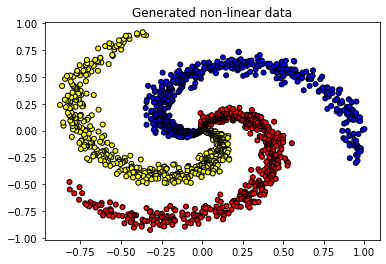### Split data

We'll shuffle our dataset (since it's ordered by class) and then create our data splits (stratified on class).

 1 2 import collections from sklearn.model_selection import train_test_split 
 1 2 3 TRAIN_SIZE = 0.7 VAL_SIZE = 0.15 TEST_SIZE = 0.15 
 1 2 3 4 5 def train_val_test_split(X, y, train_size): """Split dataset into data splits.""" X_train, X_, y_train, y_ = train_test_split(X, y, train_size=TRAIN_SIZE, stratify=y) X_val, X_test, y_val, y_test = train_test_split(X_, y_, train_size=0.5, stratify=y_) return X_train, X_val, X_test, y_train, y_val, y_test 
 1 2 3 4 5 6 7 # Create data splits X_train, X_val, X_test, y_train, y_val, y_test = train_val_test_split( X=X, y=y, train_size=TRAIN_SIZE) print (f"X_train: {X_train.shape}, y_train: {y_train.shape}") print (f"X_val: {X_val.shape}, y_val: {y_val.shape}") print (f"X_test: {X_test.shape}, y_test: {y_test.shape}") print (f"Sample point: {X_train} → {y_train}") 

X_train: (1050, 2), y_train: (1050,)
X_val: (225, 2), y_val: (225,)
X_test: (225, 2), y_test: (225,)
Sample point: [ 0.44688413 -0.07360876] → c1


### Label encoding

In the previous lesson we wrote our own label encoder class to see the inner functions but this time we'll use scikit-learn LabelEncoder class which does the same operations as ours.

 1 from sklearn.preprocessing import LabelEncoder 
 1 2 # Output vectorizer label_encoder = LabelEncoder() 
 1 2 3 4 # Fit on train data label_encoder = label_encoder.fit(y_train) classes = list(label_encoder.classes_) print (f"classes: {classes}") 

classes: ['c1', 'c2', 'c3']

 1 2 3 4 5 6 # Convert labels to tokens print (f"y_train: {y_train}") y_train = label_encoder.transform(y_train) y_val = label_encoder.transform(y_val) y_test = label_encoder.transform(y_test) print (f"y_train: {y_train}") 
y_train: c1
y_train: 0

 1 2 3 4 # Class weights counts = np.bincount(y_train) class_weights = {i: 1.0/count for i, count in enumerate(counts)} print (f"counts: {counts}\nweights: {class_weights}") 
counts: [350 350 350]
weights: {0: 0.002857142857142857, 1: 0.002857142857142857, 2: 0.002857142857142857}


### Standardize data

We need to standardize our data (zero mean and unit variance) so a specific feature's magnitude doesn't affect how the model learns its weights. We're only going to standardize the inputs X because our outputs y are class values.

 1 from sklearn.preprocessing import StandardScaler 
 1 2 # Standardize the data (mean=0, std=1) using training data X_scaler = StandardScaler().fit(X_train) 
 1 2 3 4 # Apply scaler on training and test data (don't standardize outputs for classification) X_train = X_scaler.transform(X_train) X_val = X_scaler.transform(X_val) X_test = X_scaler.transform(X_test) 
 1 2 3 # Check (means should be ~0 and std should be ~1) print (f"X_test: mean: {np.mean(X_test[:, 0], axis=0):.1f}, std: {np.std(X_test[:, 0], axis=0):.1f}") print (f"X_test: mean: {np.mean(X_test[:, 1], axis=0):.1f}, std: {np.std(X_test[:, 1], axis=0):.1f}") 

X_test: mean: -0.2, std: 0.8
X_test: mean: -0.2, std: 0.9


## Linear model

Before we get to our neural network, we're going to motivate non-linear activation functions by implementing a generalized linear model (logistic regression). We'll see why linear models (with linear activations) won't suffice for our dataset.

 1 import torch 
 1 2 # Set seed for reproducibility torch.manual_seed(SEED) 

### Model

We'll create our linear model using one layer of weights.

 1 2 from torch import nn import torch.nn.functional as F 
 1 2 3 INPUT_DIM = X_train.shape # X is 2-dimensional HIDDEN_DIM = 100 NUM_CLASSES = len(classes) # 3 classes 
  1 2 3 4 5 6 7 8 9 10 11 12 class LinearModel(nn.Module): def __init__(self, input_dim, hidden_dim, num_classes): super(LinearModel, self).__init__() self.fc1 = nn.Linear(input_dim, hidden_dim) self.fc2 = nn.Linear(hidden_dim, num_classes) def forward(self, x_in, apply_softmax=False): z = self.fc1(x_in) # linear activation y_pred = self.fc2(z) if apply_softmax: y_pred = F.softmax(y_pred, dim=1) return y_pred 
 1 2 3 # Initialize model model = LinearModel(input_dim=INPUT_DIM, hidden_dim=HIDDEN_DIM, num_classes=NUM_CLASSES) print (model.named_parameters) 

Model:



### Training

We'll go ahead and train our initialized model for a few epochs.

 1 from torch.optim import Adam 
 1 2 3 LEARNING_RATE = 1e-2 NUM_EPOCHS = 10 BATCH_SIZE = 32 
 1 2 3 # Define Loss class_weights_tensor = torch.Tensor(list(class_weights.values())) loss_fn = nn.CrossEntropyLoss(weight=class_weights_tensor) 
 1 2 3 4 5 # Accuracy def accuracy_fn(y_pred, y_true): n_correct = torch.eq(y_pred, y_true).sum().item() accuracy = (n_correct / len(y_pred)) * 100 return accuracy 
 1 2 # Optimizer optimizer = Adam(model.parameters(), lr=LEARNING_RATE) 
 1 2 3 4 5 6 7 # Convert data to tensors X_train = torch.Tensor(X_train) y_train = torch.LongTensor(y_train) X_val = torch.Tensor(X_val) y_val = torch.LongTensor(y_val) X_test = torch.Tensor(X_test) y_test = torch.LongTensor(y_test) 
  1 2 3 4 5 6 7 8 9 10 11 12 13 14 15 16 17 18 19 20 21 # Training for epoch in range(NUM_EPOCHS): # Forward pass y_pred = model(X_train) # Loss loss = loss_fn(y_pred, y_train) # Zero all gradients optimizer.zero_grad() # Backward pass loss.backward() # Update weights optimizer.step() if epoch%1==0: predictions = y_pred.max(dim=1) # class accuracy = accuracy_fn(y_pred=predictions, y_true=y_train) print (f"Epoch: {epoch} | loss: {loss:.2f}, accuracy: {accuracy:.1f}") 

Epoch: 0 | loss: 1.13, accuracy: 51.2
Epoch: 1 | loss: 0.90, accuracy: 50.0
Epoch: 2 | loss: 0.78, accuracy: 55.0
Epoch: 3 | loss: 0.74, accuracy: 54.4
Epoch: 4 | loss: 0.73, accuracy: 54.2
Epoch: 5 | loss: 0.74, accuracy: 54.7
Epoch: 6 | loss: 0.75, accuracy: 54.9
Epoch: 7 | loss: 0.75, accuracy: 54.3
Epoch: 8 | loss: 0.76, accuracy: 54.8
Epoch: 9 | loss: 0.76, accuracy: 55.0


### Evaluation

Now let's see how well our linear model does on our non-linear spiral data.

 1 2 3 import json import matplotlib.pyplot as plt from sklearn.metrics import precision_recall_fscore_support 
  1 2 3 4 5 6 7 8 9 10 11 12 13 14 15 16 17 18 19 20 21 22 def get_performance(y_true, y_pred, classes): """Per-class performance metrics.""" # Get metrics performance = {'overall': {}, 'class': {}} metrics = precision_recall_fscore_support(y_true, y_pred) # Overall performance performance['overall']['precision'] = np.mean(metrics) performance['overall']['recall'] = np.mean(metrics) performance['overall']['f1'] = np.mean(metrics) performance['overall']['num_samples'] = np.float64(np.sum(metrics)) # Per-class performance for i in range(len(classes)): performance['class'][classes[i]] = { "precision": metrics[i], "recall": metrics[i], "f1": metrics[i], "num_samples": np.float64(metrics[i]) } return performance 
 1 2 3 4 5 # Predictions y_prob = model(X_test, apply_softmax=True) print (f"sample probability: {y_prob}") y_pred = y_prob.max(dim=1) print (f"sample class: {y_pred}") 

sample probability: tensor([0.9306, 0.0683, 0.0012])
sample class: 0

 1 2 3 # Performance report performance = get_performance(y_true=y_test, y_pred=y_pred, classes=classes) print (json.dumps(performance, indent=2)) 
{
"overall": {
"precision": 0.5027661968102707,
"recall": 0.49333333333333335,
"f1": 0.4942485399571228,
"num_samples": 225.0
},
"class": {
"c1": {
"precision": 0.5068493150684932,
"recall": 0.49333333333333335,
"f1": 0.5,
"num_samples": 75.0
},
"c2": {
"precision": 0.43478260869565216,
"recall": 0.5333333333333333,
"f1": 0.47904191616766467,
"num_samples": 75.0
},
"c3": {
"precision": 0.5666666666666667,
"recall": 0.4533333333333333,
"f1": 0.5037037037037037,
"num_samples": 75.0
}
}
}


  1 2 3 4 5 6 7 8 9 10 11 12 13 14 def plot_multiclass_decision_boundary(model, X, y): x_min, x_max = X[:, 0].min() - 0.1, X[:, 0].max() + 0.1 y_min, y_max = X[:, 1].min() - 0.1, X[:, 1].max() + 0.1 xx, yy = np.meshgrid(np.linspace(x_min, x_max, 101), np.linspace(y_min, y_max, 101)) cmap = plt.cm.Spectral X_test = torch.from_numpy(np.c_[xx.ravel(), yy.ravel()]).float() y_pred = model(X_test, apply_softmax=True) _, y_pred = y_pred.max(dim=1) y_pred = y_pred.reshape(xx.shape) plt.contourf(xx, yy, y_pred, cmap=plt.cm.Spectral, alpha=0.8) plt.scatter(X[:, 0], X[:, 1], c=y, s=40, cmap=plt.cm.RdYlBu) plt.xlim(xx.min(), xx.max()) plt.ylim(yy.min(), yy.max()) 
 1 2 3 4 5 6 7 8 9 # Visualize the decision boundary plt.figure(figsize=(12,5)) plt.subplot(1, 2, 1) plt.title("Train") plot_multiclass_decision_boundary(model=model, X=X_train, y=y_train) plt.subplot(1, 2, 2) plt.title("Test") plot_multiclass_decision_boundary(model=model, X=X_test, y=y_test) plt.show()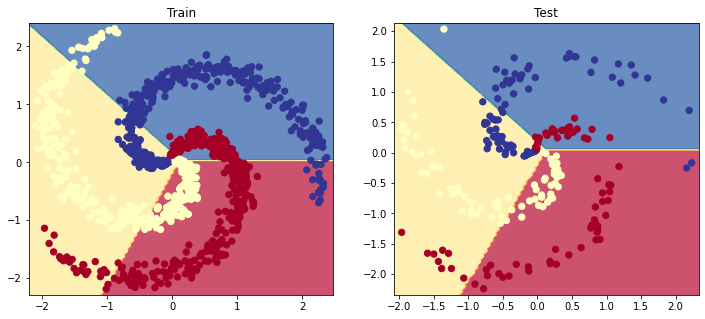## Activation functions

Using the generalized linear method (logistic regression) yielded poor results because of the non-linearity present in our data yet our activation functions were linear. We need to use an activation function that can allow our model to learn and map the non-linearity in our data. There are many different options so let's explore a few.

  1 2 3 4 5 6 7 8 9 10 11 12 13 14 15 16 17 18 19 20 21 22 23 24 25 26 # Fig size plt.figure(figsize=(12,3)) # Data x = torch.arange(-5., 5., 0.1) # Sigmoid activation (constrain a value between 0 and 1.) plt.subplot(1, 3, 1) plt.title("Sigmoid activation") y = torch.sigmoid(x) plt.plot(x.numpy(), y.numpy()) # Tanh activation (constrain a value between -1 and 1.) plt.subplot(1, 3, 2) y = torch.tanh(x) plt.title("Tanh activation") plt.plot(x.numpy(), y.numpy()) # Relu (clip the negative values to 0) plt.subplot(1, 3, 3) y = F.relu(x) plt.title("ReLU activation") plt.plot(x.numpy(), y.numpy()) # Show plots plt.show()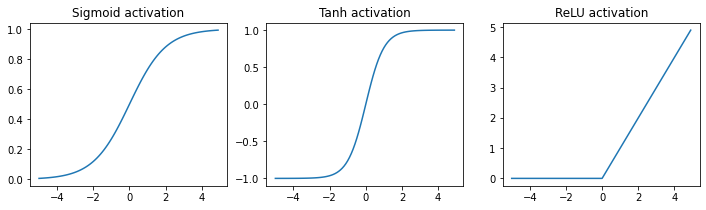The ReLU activation function ($$max(0,z)$$) is by far the most widely used activation function for neural networks. But as you can see, each activation function has its own constraints so there are circumstances where you'll want to use different ones. For example, if we need to constrain our outputs between 0 and 1, then the sigmoid activation is the best choice.

Note

In some cases, using a ReLU activation function may not be sufficient. For instance, when the outputs from our neurons are mostly negative, the activation function will produce zeros. This effectively creates a "dying ReLU" and a recovery is unlikely. To mitigate this effect, we could lower the learning rate or use alternative ReLU activations, ex. leaky ReLU or parametric ReLU (PReLU), which have a small slope for negative neuron outputs.

## NumPy

Now let's create our multilayer perceptron (MLP) which is going to be exactly like the logistic regression model but with the activation function to map the non-linearity in our data.

Note

It's normal to find the math and code in this section slightly complex. You can still read each of the steps to build intuition for when we implement this using PyTorch.

Our goal is to learn a model $$\hat{y}$$ that models $$y$$ given $$X$$. You'll notice that neural networks are just extensions of the generalized linear methods we've seen so far but with non-linear activation functions since our data will be highly non-linear.

$z_1 = XW_1$
$a_1 = f(z_1)$
$z_2 = a_1W_2$
$\hat{y} = softmax(z_2)$

### Initialize weights

Step 1: Randomly initialize the model's weights $$W$$ (we'll cover more effective initialization strategies later in this lesson).

 1 2 3 4 5 # Initialize first layer's weights W1 = 0.01 * np.random.randn(INPUT_DIM, HIDDEN_DIM) b1 = np.zeros((1, HIDDEN_DIM)) print (f"W1: {W1.shape}") print (f"b1: {b1.shape}") 

W1: (2, 100)
b1: (1, 100)


### Model

Step 2: Feed inputs $$X$$ into the model to do the forward pass and receive the probabilities. First we pass the inputs into the first layer.

$z_1 = XW_1$
 1 2 3 # z1 = [NX2] · [2X100] + [1X100] = [NX100] z1 = np.dot(X_train, W1) + b1 print (f"z1: {z1.shape}") 
z1: (1050, 100)


Next we apply the non-linear activation function, ReLU ($$max(0,z)$$) in this case.

$a_1 = f(z_1)$
 1 2 3 # Apply activation function a1 = np.maximum(0, z1) # ReLU print (f"a_1: {a1.shape}") 
a_1: (1050, 100)


We pass the activations to the second layer to get our logits.

$z_2 = a_1W_2$
 1 2 3 4 5 # Initialize second layer's weights W2 = 0.01 * np.random.randn(HIDDEN_DIM, NUM_CLASSES) b2 = np.zeros((1, NUM_CLASSES)) print (f"W2: {W2.shape}") print (f"b2: {b2.shape}") 
W2: (100, 3)
b2: (1, 3)

 1 2 3 4 # z2 = logits = [NX100] · [100X3] + [1X3] = [NX3] logits = np.dot(a1, W2) + b2 print (f"logits: {logits.shape}") print (f"sample: {logits}") 
logits: (1050, 3)
sample: [-9.85444376e-05  1.67334360e-03 -6.31717987e-04]


We'll apply the softmax function to normalize the logits and btain class probabilities.

$\hat{y} = softmax(z_2)$
 1 2 3 4 5 # Normalization via softmax to obtain class probabilities exp_logits = np.exp(logits) y_hat = exp_logits / np.sum(exp_logits, axis=1, keepdims=True) print (f"y_hat: {y_hat.shape}") print (f"sample: {y_hat}") 
y_hat: (1050, 3)
sample: [0.33319557 0.33378647 0.33301796]


### Loss

Step 3: Compare the predictions $$\hat{y}$$ (ex. [0.3, 0.3, 0.4]) with the actual target values $$y$$ (ex. class 2 would look like [0, 0, 1]) with the objective (cost) function to determine loss $$J$$. A common objective function for classification tasks is cross-entropy loss.

$J(\theta) = - \sum_i ln(\hat{y_i}) = - \sum_i ln (\frac{e^{X_iW_y}}{\sum_j e^{X_iW}})$
 1 2 3 4 # Loss correct_class_logprobs = -np.log(y_hat[range(len(y_hat)), y_train]) loss = np.sum(correct_class_logprobs) / len(y_train) print (f"loss: {loss:.2f}") 
loss: 0.70


Step 4: Calculate the gradient of loss $$J(\theta)$$ w.r.t to the model weights.

The gradient of the loss w.r.t to $$W_2$$ is the same as the gradients from logistic regression since \$$$hat{y} = softmax(z_2)$$.

$\frac{\partial{J}}{\partial{W_{2j}}} = \frac{\partial{J}}{\partial{\hat{y}}}\frac{\partial{\hat{y}}}{\partial{W_{2j}}} = - \frac{1}{\hat{y}}\frac{\partial{\hat{y}}}{\partial{W_{2j}}} =$
$= - \frac{1}{\frac{e^{W_{2y}a_1}}{\sum_j e^{a_1W}}}\frac{\sum_j e^{a_1W}e^{a_1W_{2y}}0 - e^{a_1W_{2y}}e^{a_1W_{2j}}a_1}{(\sum_j e^{a_1W})^2} = \frac{a_1e^{a_1W_{2j}}}{\sum_j e^{a_1W}} = a_1\hat{y}$
$\frac{\partial{J}}{\partial{W_{2y}}} = \frac{\partial{J}}{\partial{\hat{y}}}\frac{\partial{\hat{y}}}{\partial{W_{2y}}} = - \frac{1}{\hat{y}}\frac{\partial{\hat{y}}}{\partial{W_{2y}}} =$
$= - \frac{1}{\frac{e^{W_{2y}a_1}}{\sum_j e^{a_1W}}}\frac{\sum_j e^{a_1W}e^{a_1W_{2y}}a_1 - e^{a_1W_{2y}}e^{a_1W_{2y}}a_1}{(\sum_j e^{a_1W})^2} = \frac{1}{\hat{y}}(a_1\hat{y} - a_1\hat{y}^2) = a_1(\hat{y}-1)$

The gradient of the loss w.r.t $$W_1$$ is a bit trickier since we have to backpropagate through two sets of weights.

$\frac{\partial{J}}{\partial{W_1}} = \frac{\partial{J}}{\partial{\hat{y}}} \frac{\partial{\hat{y}}}{\partial{a_1}} \frac{\partial{a_1}}{\partial{z_1}} \frac{\partial{z_1}}{\partial{W_1}} = W_2(\partial{scores})(\partial{ReLU})X$

 1 2 3 4 5 6 # dJ/dW2 dscores = y_hat dscores[range(len(y_hat)), y_train] -= 1 dscores /= len(y_train) dW2 = np.dot(a1.T, dscores) db2 = np.sum(dscores, axis=0, keepdims=True) 
 1 2 3 4 5 # dJ/dW1 dhidden = np.dot(dscores, W2.T) dhidden[a1 <= 0] = 0 # ReLu backprop dW1 = np.dot(X_train.T, dhidden) db1 = np.sum(dhidden, axis=0, keepdims=True) 

### Update weights

Step 5: Update the weights $$W$$ using a small learning rate $$\alpha$$. The updates will penalize the probability for the incorrect classes ($$j $$\() and encourage a higher probability for the correct class ($$\) y$$).

$W_i = W_i - \alpha\frac{\partial{J}}{\partial{W_i}}$
 1 2 3 4 5 # Update weights W1 += -LEARNING_RATE * dW1 b1 += -LEARNING_RATE * db1 W2 += -LEARNING_RATE * dW2 b2 += -LEARNING_RATE * db2 

### Training

Step 6: Repeat steps 2 - 4 until model performs well.

 1 2 3 4 5 6 7 # Convert tensors to NumPy arrays X_train = X_train.numpy() y_train = y_train.numpy() X_val = X_val.numpy() y_val = y_val.numpy() X_test = X_test.numpy() y_test = y_test.numpy() 
  1 2 3 4 5 6 7 8 9 10 11 12 13 14 15 16 17 18 19 20 21 22 23 24 25 26 27 28 29 30 31 32 33 34 35 36 37 38 39 40 41 42 43 44 45 46 47 48 49 50 51 # Initialize random weights W1 = 0.01 * np.random.randn(INPUT_DIM, HIDDEN_DIM) b1 = np.zeros((1, HIDDEN_DIM)) W2 = 0.01 * np.random.randn(HIDDEN_DIM, NUM_CLASSES) b2 = np.zeros((1, NUM_CLASSES)) # Training loop for epoch_num in range(1000): # First layer forward pass [NX2] · [2X100] = [NX100] z1 = np.dot(X_train, W1) + b1 # Apply activation function a1 = np.maximum(0, z1) # ReLU # z2 = logits = [NX100] · [100X3] = [NX3] logits = np.dot(a1, W2) + b2 # Normalization via softmax to obtain class probabilities exp_logits = np.exp(logits) y_hat = exp_logits / np.sum(exp_logits, axis=1, keepdims=True) # Loss correct_class_logprobs = -np.log(y_hat[range(len(y_hat)), y_train]) loss = np.sum(correct_class_logprobs) / len(y_train) # show progress if epoch_num%100 == 0: # Accuracy y_pred = np.argmax(logits, axis=1) accuracy = np.mean(np.equal(y_train, y_pred)) print (f"Epoch: {epoch_num}, loss: {loss:.3f}, accuracy: {accuracy:.3f}") # dJ/dW2 dscores = y_hat dscores[range(len(y_hat)), y_train] -= 1 dscores /= len(y_train) dW2 = np.dot(a1.T, dscores) db2 = np.sum(dscores, axis=0, keepdims=True) # dJ/dW1 dhidden = np.dot(dscores, W2.T) dhidden[a1 <= 0] = 0 # ReLu backprop dW1 = np.dot(X_train.T, dhidden) db1 = np.sum(dhidden, axis=0, keepdims=True) # Update weights W1 += -1e0 * dW1 b1 += -1e0 * db1 W2 += -1e0 * dW2 b2 += -1e0 * db2 

Epoch: 0, loss: 1.099, accuracy: 0.339
Epoch: 100, loss: 0.549, accuracy: 0.678
Epoch: 200, loss: 0.238, accuracy: 0.907
Epoch: 300, loss: 0.151, accuracy: 0.946
Epoch: 400, loss: 0.098, accuracy: 0.972
Epoch: 500, loss: 0.074, accuracy: 0.985
Epoch: 600, loss: 0.059, accuracy: 0.988
Epoch: 700, loss: 0.050, accuracy: 0.991
Epoch: 800, loss: 0.043, accuracy: 0.992
Epoch: 900, loss: 0.038, accuracy: 0.993


### Evaluation

Now let's see how our model performs on the test (hold-out) data split.

 1 2 3 4 5 6 7 8 class MLPFromScratch(): def predict(self, x): z1 = np.dot(x, W1) + b1 a1 = np.maximum(0, z1) logits = np.dot(a1, W2) + b2 exp_logits = np.exp(logits) y_hat = exp_logits / np.sum(exp_logits, axis=1, keepdims=True) return y_hat 
 1 2 3 4 # Evaluation model = MLPFromScratch() y_prob = model.predict(X_test) y_pred = np.argmax(y_prob, axis=1) 
 1 2 3 # Performance report performance = get_performance(y_true=y_test, y_pred=y_pred, classes=classes) print (json.dumps(performance, indent=2)) 

{
"overall": {
"precision": 0.9824531024531025,
"recall": 0.9822222222222222,
"f1": 0.982220641694326,
"num_samples": 225.0
},
"class": {
"c1": {
"precision": 1.0,
"recall": 0.9733333333333334,
"f1": 0.9864864864864865,
"num_samples": 75.0
},
"c2": {
"precision": 0.974025974025974,
"recall": 1.0,
"f1": 0.9868421052631579,
"num_samples": 75.0
},
"c3": {
"precision": 0.9733333333333334,
"recall": 0.9733333333333334,
"f1": 0.9733333333333334,
"num_samples": 75.0
}
}
}


  1 2 3 4 5 6 7 8 9 10 11 12 13 14 15 16 17 18 19 20 21 22 23 24 25 26 27 28 29 def plot_multiclass_decision_boundary_numpy(model, X, y, savefig_fp=None): """Plot the multiclass decision boundary for a model that accepts 2D inputs. Credit: https://cs231n.github.io/neural-networks-case-study/ Arguments: model {function} -- trained model with function model.predict(x_in). X {numpy.ndarray} -- 2D inputs with shape (N, 2). y {numpy.ndarray} -- 1D outputs with shape (N,). """ # Axis boundaries x_min, x_max = X[:, 0].min() - 0.1, X[:, 0].max() + 0.1 y_min, y_max = X[:, 1].min() - 0.1, X[:, 1].max() + 0.1 xx, yy = np.meshgrid(np.linspace(x_min, x_max, 101), np.linspace(y_min, y_max, 101)) # Create predictions x_in = np.c_[xx.ravel(), yy.ravel()] y_pred = model.predict(x_in) y_pred = np.argmax(y_pred, axis=1).reshape(xx.shape) # Plot decision boundary plt.contourf(xx, yy, y_pred, cmap=plt.cm.Spectral, alpha=0.8) plt.scatter(X[:, 0], X[:, 1], c=y, s=40, cmap=plt.cm.RdYlBu) plt.xlim(xx.min(), xx.max()) plt.ylim(yy.min(), yy.max()) # Plot if savefig_fp: plt.savefig(savefig_fp, format='png') 
 1 2 3 4 5 6 7 8 9 # Visualize the decision boundary plt.figure(figsize=(12,5)) plt.subplot(1, 2, 1) plt.title("Train") plot_multiclass_decision_boundary_numpy(model=model, X=X_train, y=y_train) plt.subplot(1, 2, 2) plt.title("Test") plot_multiclass_decision_boundary_numpy(model=model, X=X_test, y=y_test) plt.show()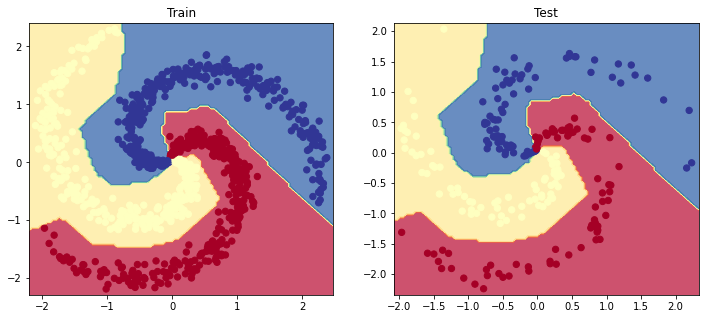## PyTorch

Now let's implement the same MLP in PyTorch.

### Model

We'll be using two linear layers along with PyTorch Functional API's ReLU operation.

  1 2 3 4 5 6 7 8 9 10 11 12 class MLP(nn.Module): def __init__(self, input_dim, hidden_dim, num_classes): super(MLP, self).__init__() self.fc1 = nn.Linear(input_dim, hidden_dim) self.fc2 = nn.Linear(hidden_dim, num_classes) def forward(self, x_in, apply_softmax=False): z = F.relu(self.fc1(x_in)) # ReLU activaton function added! y_pred = self.fc2(z) if apply_softmax: y_pred = F.softmax(y_pred, dim=1) return y_pred 
 1 2 3 # Initialize model model = MLP(input_dim=INPUT_DIM, hidden_dim=HIDDEN_DIM, num_classes=NUM_CLASSES) print (model.named_parameters) 




### Training

 1 2 3 # Define Loss class_weights_tensor = torch.Tensor(list(class_weights.values())) loss_fn = nn.CrossEntropyLoss(weight=class_weights_tensor) 
 1 2 3 4 5 # Accuracy def accuracy_fn(y_pred, y_true): n_correct = torch.eq(y_pred, y_true).sum().item() accuracy = (n_correct / len(y_pred)) * 100 return accuracy 
 1 2 # Optimizer optimizer = Adam(model.parameters(), lr=LEARNING_RATE) 
 1 2 3 4 5 6 7 # Convert data to tensors X_train = torch.Tensor(X_train) y_train = torch.LongTensor(y_train) X_val = torch.Tensor(X_val) y_val = torch.LongTensor(y_val) X_test = torch.Tensor(X_test) y_test = torch.LongTensor(y_test) 
  1 2 3 4 5 6 7 8 9 10 11 12 13 14 15 16 17 18 19 20 21 # Training for epoch in range(NUM_EPOCHS*10): # Forward pass y_pred = model(X_train) # Loss loss = loss_fn(y_pred, y_train) # Zero all gradients optimizer.zero_grad() # Backward pass loss.backward() # Update weights optimizer.step() if epoch%10==0: predictions = y_pred.max(dim=1) # class accuracy = accuracy_fn(y_pred=predictions, y_true=y_train) print (f"Epoch: {epoch} | loss: {loss:.2f}, accuracy: {accuracy:.1f}") 

Epoch: 0 | loss: 1.11, accuracy: 21.9
Epoch: 10 | loss: 0.66, accuracy: 59.8
Epoch: 20 | loss: 0.50, accuracy: 73.0
Epoch: 30 | loss: 0.38, accuracy: 89.8
Epoch: 40 | loss: 0.28, accuracy: 92.3
Epoch: 50 | loss: 0.21, accuracy: 93.8
Epoch: 60 | loss: 0.17, accuracy: 95.2
Epoch: 70 | loss: 0.14, accuracy: 96.1
Epoch: 80 | loss: 0.12, accuracy: 97.4
Epoch: 90 | loss: 0.10, accuracy: 97.8


### Evaluation

 1 2 3 # Predictions y_prob = model(X_test, apply_softmax=True) y_pred = y_prob.max(dim=1) 
 1 2 3 # Performance report performance = get_performance(y_true=y_test, y_pred=y_pred, classes=classes) print (json.dumps(performance, indent=2)) 

{
"overall": {
"precision": 0.9706790123456791,
"recall": 0.9688888888888889,
"f1": 0.9690388976103262,
"num_samples": 225.0
},
"class": {
"c1": {
"precision": 1.0,
"recall": 0.96,
"f1": 0.9795918367346939,
"num_samples": 75.0
},
"c2": {
"precision": 0.9259259259259259,
"recall": 1.0,
"f1": 0.9615384615384615,
"num_samples": 75.0
},
"c3": {
"precision": 0.9861111111111112,
"recall": 0.9466666666666667,
"f1": 0.9659863945578231,
"num_samples": 75.0
}
}
}

 1 2 3 4 5 6 7 8 9 # Visualize the decision boundary plt.figure(figsize=(12,5)) plt.subplot(1, 2, 1) plt.title("Train") plot_multiclass_decision_boundary(model=model, X=X_train, y=y_train) plt.subplot(1, 2, 2) plt.title("Test") plot_multiclass_decision_boundary(model=model, X=X_test, y=y_test) plt.show()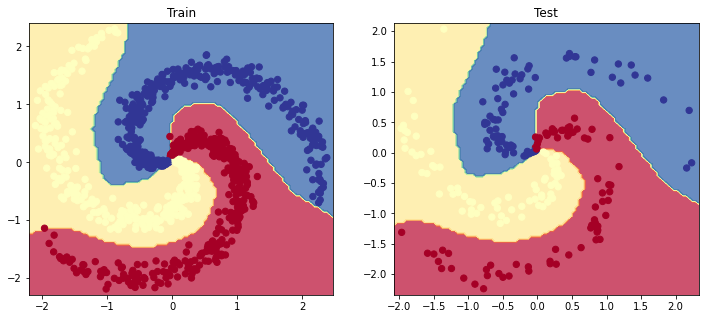### Inference

Let's look at the inference operations when using our trained model.

 1 2 # Inputs for inference X_infer = pd.DataFrame([{'X1': 0.1, 'X2': 0.1}]) 
 1 2 3 # Standardize X_infer = X_scaler.transform(X_infer) print (X_infer) 

[[0.22746497 0.29242354]]

 1 2 3 4 5 # Predict y_infer = model(torch.Tensor(X_infer), apply_softmax=True) prob, _class = y_infer.max(dim=1) label = label_encoder.inverse_transform(_class.detach().numpy()) print (f"The probability that you have {label} is {prob.detach().numpy()*100.0:.0f}%") 
The probability that you have c1 is 92%


## Initializing weights

So far we have been initializing weights with small random values and this isn't optimal for convergence during training. The objective is to have weights that are able to produce outputs that follow a similar distribution across all neurons. We can do this by enforcing weights to have unit variance prior the affine and non-linear operations.

Note

A popular method is to apply xavier initialization, which essentially initializes the weights to allow the signal from the data to reach deep into the network. You may be wondering why we don't do this for every forward pass and that's a great question. We'll look at more advanced strategies that help with optimization like batch/layer normalization, etc. in future lessons. Meanwhile you can check out other initializers here.

 1 from torch.nn import init 
  1 2 3 4 5 6 7 8 9 10 11 12 13 14 15 class MLP(nn.Module): def __init__(self, input_dim, hidden_dim, num_classes): super(MLP, self).__init__() self.fc1 = nn.Linear(input_dim, hidden_dim) self.fc2 = nn.Linear(hidden_dim, num_classes) def init_weights(self): init.xavier_normal(self.fc1.weight, gain=init.calculate_gain('relu')) def forward(self, x_in, apply_softmax=False): z = F.relu(self.fc1(x_in)) # ReLU activaton function added! y_pred = self.fc2(z) if apply_softmax: y_pred = F.softmax(y_pred, dim=1) return y_pred 

## Dropout

A great technique to have our models generalize (perform well on test data) is to increase the size of your data but this isn't always an option. Fortuntely, there are methods like regularization and dropout that can help create a more robust model.

Dropout is a technique (used only during training) that allows us to zero the outputs of neurons. We do this for dropout_p% of the total neurons in each layer and it changes every batch. Dropout prevents units from co-adapting too much to the data and acts as a sampling strategy since we drop a different set of neurons each time.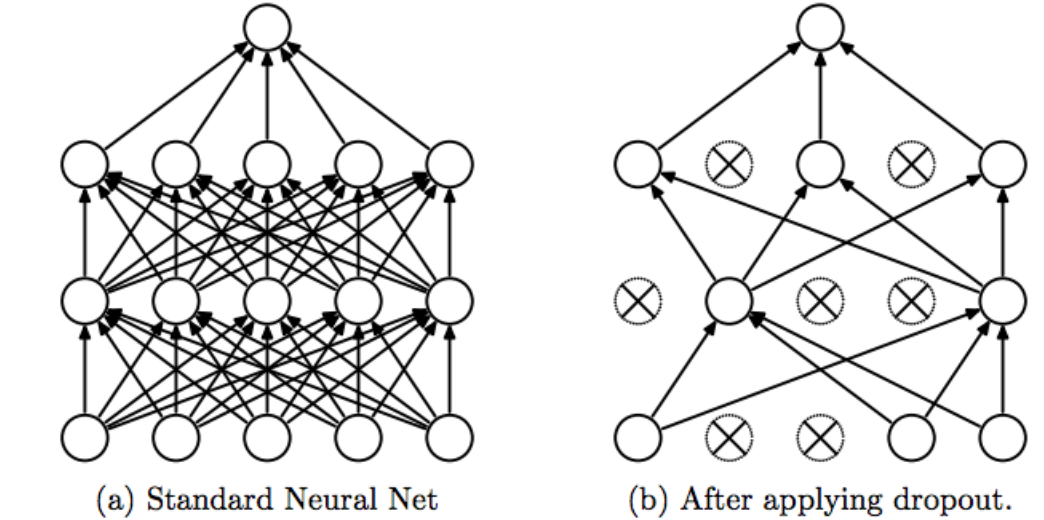1 DROPOUT_P = 0.1 # percetange of weights that are dropped each pass 
  1 2 3 4 5 6 7 8 9 10 11 12 13 14 15 16 17 class MLP(nn.Module): def __init__(self, input_dim, hidden_dim, dropout_p, num_classes): super(MLP, self).__init__() self.fc1 = nn.Linear(input_dim, hidden_dim) self.dropout = nn.Dropout(dropout_p) # dropout self.fc2 = nn.Linear(hidden_dim, num_classes) def init_weights(self): init.xavier_normal(self.fc1.weight, gain=init.calculate_gain('relu')) def forward(self, x_in, apply_softmax=False): z = F.relu(self.fc1(x_in)) z = self.dropout(z) # dropout y_pred = self.fc2(z) if apply_softmax: y_pred = F.softmax(y_pred, dim=1) return y_pred 
 1 2 3 4 # Initialize model model = MLP(input_dim=INPUT_DIM, hidden_dim=HIDDEN_DIM, dropout_p=DROPOUT_P, num_classes=NUM_CLASSES) print (model.named_parameters) 




## Overfitting

Though neural networks are great at capturing non-linear relationships they are highly susceptible to overfitting to the training data and failing to generalize on test data. Just take a look at the example below where we generate completely random data and are able to fit a model with $$2*N*C + D$$ hidden units. The training performance is good (~70%) but the overfitting leads to very poor test performance. We'll be covering strategies to tackle overfitting in future lessons.

 1 2 3 4 NUM_EPOCHS = 500 NUM_SAMPLES_PER_CLASS = 50 LEARNING_RATE = 1e-1 HIDDEN_DIM = 2 * NUM_SAMPLES_PER_CLASS * NUM_CLASSES + INPUT_DIM # 2*N*C + D 
 1 2 3 4 5 # Generate random data X = np.random.rand(NUM_SAMPLES_PER_CLASS * NUM_CLASSES, INPUT_DIM) y = np.array([[i]*NUM_SAMPLES_PER_CLASS for i in range(NUM_CLASSES)]).reshape(-1) print ("X: ", format(np.shape(X))) print ("y: ", format(np.shape(y))) 

X:  (150, 2)
y:  (150,)

 1 2 3 4 5 6 7 # Create data splits X_train, X_val, X_test, y_train, y_val, y_test = train_val_test_split( X=X, y=y, train_size=TRAIN_SIZE) print (f"X_train: {X_train.shape}, y_train: {y_train.shape}") print (f"X_val: {X_val.shape}, y_val: {y_val.shape}") print (f"X_test: {X_test.shape}, y_test: {y_test.shape}") print (f"Sample point: {X_train} → {y_train}") 
X_train: (105, 2), y_train: (105,)
X_val: (22, 2), y_val: (22,)
X_test: (23, 2), y_test: (23,)
Sample point: [0.52553355 0.33956916] → 0


 1 2 3 4 5 # Standardize the inputs (mean=0, std=1) using training data X_scaler = StandardScaler().fit(X_train) X_train = X_scaler.transform(X_train) X_val = X_scaler.transform(X_val) X_test = X_scaler.transform(X_test) 
 1 2 3 4 5 6 7 # Convert data to tensors X_train = torch.Tensor(X_train) y_train = torch.LongTensor(y_train) X_val = torch.Tensor(X_val) y_val = torch.LongTensor(y_val) X_test = torch.Tensor(X_test) y_test = torch.LongTensor(y_test) 
 1 2 3 4 # Initialize model model = MLP(input_dim=INPUT_DIM, hidden_dim=HIDDEN_DIM, dropout_p=DROPOUT_P, num_classes=NUM_CLASSES) print (model.named_parameters) 




 1 2 # Optimizer optimizer = Adam(model.parameters(), lr=LEARNING_RATE) 
  1 2 3 4 5 6 7 8 9 10 11 12 13 14 15 16 17 18 19 20 21 # Training for epoch in range(NUM_EPOCHS): # Forward pass y_pred = model(X_train) # Loss loss = loss_fn(y_pred, y_train) # Zero all gradients optimizer.zero_grad() # Backward pass loss.backward() # Update weights optimizer.step() if epoch%20==0: predictions = y_pred.max(dim=1) # class accuracy = accuracy_fn(y_pred=predictions, y_true=y_train) print (f"Epoch: {epoch} | loss: {loss:.2f}, accuracy: {accuracy:.1f}") 

Epoch: 0 | loss: 1.15, accuracy: 37.1
Epoch: 20 | loss: 1.04, accuracy: 47.6
Epoch: 40 | loss: 0.98, accuracy: 51.4
Epoch: 60 | loss: 0.90, accuracy: 57.1
Epoch: 80 | loss: 0.87, accuracy: 59.0
Epoch: 100 | loss: 0.88, accuracy: 58.1
Epoch: 120 | loss: 0.84, accuracy: 64.8
Epoch: 140 | loss: 0.86, accuracy: 61.0
Epoch: 160 | loss: 0.81, accuracy: 64.8
Epoch: 180 | loss: 0.89, accuracy: 59.0
Epoch: 200 | loss: 0.91, accuracy: 60.0
Epoch: 220 | loss: 0.82, accuracy: 63.8
Epoch: 240 | loss: 0.86, accuracy: 59.0
Epoch: 260 | loss: 0.77, accuracy: 66.7
Epoch: 280 | loss: 0.82, accuracy: 67.6
Epoch: 300 | loss: 0.88, accuracy: 57.1
Epoch: 320 | loss: 0.81, accuracy: 61.9
Epoch: 340 | loss: 0.79, accuracy: 63.8
Epoch: 360 | loss: 0.80, accuracy: 61.0
Epoch: 380 | loss: 0.86, accuracy: 64.8
Epoch: 400 | loss: 0.77, accuracy: 64.8
Epoch: 420 | loss: 0.79, accuracy: 64.8
Epoch: 440 | loss: 0.81, accuracy: 65.7
Epoch: 460 | loss: 0.77, accuracy: 70.5
Epoch: 480 | loss: 0.80, accuracy: 67.6


 1 2 3 # Predictions y_prob = model(X_test, apply_softmax=True) y_pred = y_prob.max(dim=1) 
 1 2 3 # Performance report performance = get_performance(y_true=y_test, y_pred=y_pred, classes=classes) print (json.dumps(performance, indent=2)) 

{
"overall": {
"precision": 0.17857142857142858,
"recall": 0.16666666666666666,
"f1": 0.1722222222222222,
"num_samples": 23.0
},
"class": {
"c1": {
"precision": 0.0,
"recall": 0.0,
"f1": 0.0,
"num_samples": 7.0
},
"c2": {
"precision": 0.2857142857142857,
"recall": 0.25,
"f1": 0.26666666666666666,
"num_samples": 8.0
},
"c3": {
"precision": 0.25,
"recall": 0.25,
"f1": 0.25,
"num_samples": 8.0
}
}
}

 1 2 3 4 5 6 7 8 9 # Visualize the decision boundary plt.figure(figsize=(12,5)) plt.subplot(1, 2, 1) plt.title("Train") plot_multiclass_decision_boundary(model=model, X=X_train, y=y_train) plt.subplot(1, 2, 2) plt.title("Test") plot_multiclass_decision_boundary(model=model, X=X_test, y=y_test) plt.show()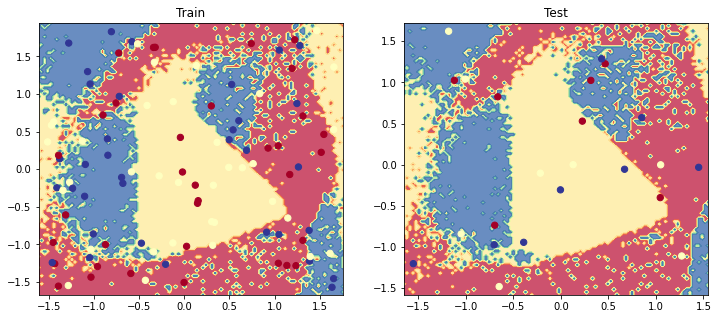It's important that we experiment, starting with simple models that underfit (high bias) and improve it towards a good fit. Starting with simple models (linear/logistic regression) let's us catch errors without the added complexity of more sophisticated models (neural networks).